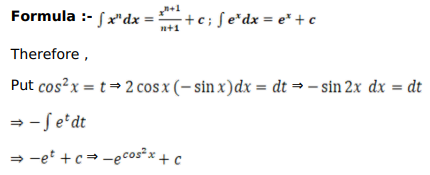# Mark against the correct answer in each of the following:

Question:

Mark $(\sqrt{ })$ against the correct answer in each of the following:

$\int e^{\cos ^{2} x} \sin 2 x d x=?$

A. $e^{\cos ^{2} x}+C$

B. $-e^{\cos ^{2} x}+C$

C. $e^{\sin ^{2} x}+C$

D. none of these

Solution: